The rule for division by the reciprocal methodCustom SearchThe rule for division by the reciprocal method is: Multiply the dividend by the reciprocal of the divisor. This is sometimes stated in short form as follows: Invert the divisor and multiply. The following examples of cases that arise in division with fractions will be solved by both the reciprocal method and the common denominator method. The common denominator method more clearly shows the division process and is easier for the beginner to grasp. The reciprocal method is more obscure as to the reason for its use but has the advantage of speed and the possibility of cancellation of like factors, which simplifies the computation. It is the suggested method once the principles become familiar . EXAMPLE:Common Denominator         Method                             Reciprocal MethodEXAMPLE:Common Denominator         Method                             Reciprocal Method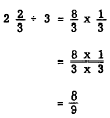EXAMPLE:Common Denominator         Method                                 Reciprocal Method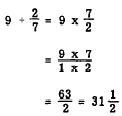EXAMPLE: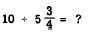Common Denominator         Method                     Reciprocal Method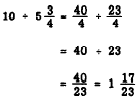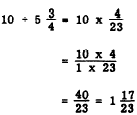EXAMPLE:Common Denominator         Method                     Reciprocal Method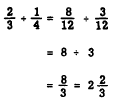EXAMPLE: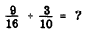Common Denominator         Method                    Reciprocal Method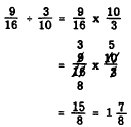Practice problems. Perform the following division by the reciprocal method: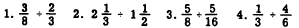Answers: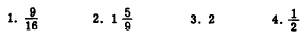COMPLEX FRACTIONS When the numerator or denominator, or both, in a fraction are themselves composed of fractions, the resulting expression is called a complex fraction. The following expression is a complex fraction: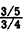This should be read "three-fifths over three-fourths" or "three-fifths divided by three-fourths," Any complex fraction may be simplified by writing it as a division problem, as follows:Similarly,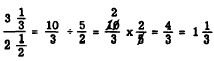Complex fractions may also contain an indicated operation in the numerator or denominator or both. Thus,is a complex fraction. To simplify such a fraction we simplify the numerator and denominator and proceed as follows:Mixed numbers appearing in complex fractions usually show the plus sign. Thus,might be writtenPractice problems. Simplify the following complex fractions: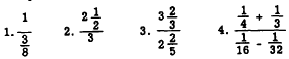Answers:Complex fractions may arise in electronics when it is necessary to find the total resistance of several resistances in parallel as shown in figure 4-8. The rule is: The total resistance of a parallel circuit is 1 divided by the sum of the reciprocals of the separate resistances. Written as a formula, this produces the following expression: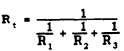EXAMPLE: Find the total resistance of the parallel circuit in figure 4-8 (A). Substituting the values 3, 4, and 6 for the letters R1, R2, and R3, we have the following.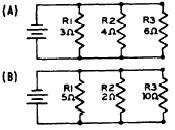Figure 4-8.-Application of complex fractions in calculating electrical resistance.The LCD of the fractions 1/3, 1/4 and 1/6 is 12. Thus,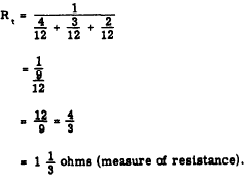Practice problem: Find the total resistance of the parallel circuit in figure 4-8 (B). Answer: 1 1/4 ohms.Integrated Publishing, Inc. - A (SDVOSB) Service Disabled Veteran Owned Small Business